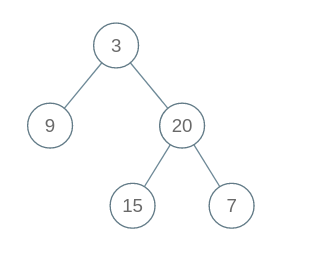# Construct Binary Tree from Inorder and Postorder Traversal

Posted: 22 Mar, 2021
Difficulty: Moderate

## PROBLEM STATEMENT

#### Ninja has been given a postorder and inorder traversal of a Binary Tree of type integer with ‘N’ nodes stored in an array/list ‘POST_ ORDER’ and ‘IN_ORDER’. Ninja has to create the binary tree using the given two arrays/lists and return the root of that tree.

##### Note:
``````Assume that the Binary Tree contains only unique elements.
``````
##### For example:
``````Let's assume: ‘IN_ORDER’ = [9, 3, 15, 20, 7]  and ‘POST_ORDER’= [9, 15, 7, 20, 3].
We get the following binary tree from Inorder and Postorder traversal:
``````##### Input Format
``````The first line of input contains an integer ‘T’ which denotes the number of test cases or queries to be run. Then the test cases follow.

The first line of each test case contains an integer ‘N’ which represents the number of nodes in the Binary Tree.

The next line of each test case contains ‘N’ single space-separated integers, representing the Postorder traversal of the Binary Tree.

The next line of each test case contains ‘N’ single space-separated integers, representing the Inorder traversal of the Binary Tree.
``````
##### Output Format :
``````For each test case, print the level order traversal of the Binary Tree.

Print the output of each test case in a separate line.
``````
##### Note:
``````You do not need to print anything; it has already been taken care of. Just implement the given function.
``````
##### Constraints :
``````1 <= ‘T’ <= 10
1 <= ‘N’ <= 10000
1 <=  ‘POST_ORDER[i]’ , ‘IN_ORDER[i]’ <= 100000

Where ‘T’ denotes the number of test cases, ‘N’ denotes the number of nodes in the tree, and  ‘POST_ORDER[i]’ and ‘IN_ORDER[i]’ represent the value of the node in Postorder-traversal and Inorder-traversal of the Binary Tree respectively.

Time Limit: 1 second
``````Approach 1

To solve this problem, first, we take the last element in the ‘POST_ORDER’  array/list as the root because we know post-order traversal is like the first traverse of the left subtree nodes and then the right subtree node at the end traverse root. Hence the root is present as the last element of post-order traversal. Then we find the position of the root data in the ‘IN_ORDER’ array/list. Let’s assume at the ‘Kth’ index we find the root data in the ‘IN_ORDER’ array/list. Then we locate the range for the left subtree and right subtree and recursively call the function for finding the left subtree and the right subtree.

For example:

First, we select the last element of the ‘POST_ORDER’  array/list as a root. Then we find the 3 in the ‘IN_ORDER’ array/list. All the elements to the left of 3 in the ‘IN_ORDER’ array/list will be part of the left subtree and all the elements to the right of 3 in ‘IN_ORDER’ will be part of the right subtree as highlighted above.

The number of elements on the left side of the ‘IN_ORDER’ array/list is 1 in our example. So, 1 element in the ‘POST_ORDER’  array/list from starting will be part of the left subtree and the next elements less than the root index will be part of the right subtree.

Here is the algorithm:

1. We declare four variables ‘POST_START’, ‘POST_END’, ‘IN_START’, and ‘IN_END’ representing the starting and ending position of ‘POST_ORDER’  and ‘IN_ORDER’ respectively.
2. Base case: If  ‘POST_START’ is greater than ‘POST_END’ or ‘IN_START’ is greater than ‘IN_END’:
• Return ‘NULL’.
3. We declare a variable ‘ROOT_VAL’ which is equal to ‘POST_ORDER[‘POST_END’]’.
4. We make a Binary Tree node using ‘ROOT_VAL’ as data of the node.
5. We declare a variable ‘ROOT_INDEX’ in which we store the index of root value that is present in the ‘IN_ORDER’ array/list.
6. We run a loop for ‘i’ = ‘IN_START’ to ‘IN_END’:
• If ‘ROOT_VAL’ is equal to ‘IN_ORDER[i]’:
• ‘ROOT_INDEX’ is equal to ‘i’.
• Break.
7. Now, the left subtree ‘IN_END’ is ‘ROOT_INDEX’ - 1 and ‘POST_END’ is ‘POST_START’ + ROOT_INDEX’ - 1 - ‘IN_START’.
8. The right subtree ‘IN_START’ is ‘ROOT_INDEX’ + 1 and ‘POST_START’ is ‘POST_START’ + ‘ROOT_INDEX’  - ‘IN_START’ and  ‘POST_END’ is ‘POST_START’ - 1.
9. Now, we use recursion for finding the left subtree and the right subtree.
10. Finally, we return the ‘ROOT’ of the Binary Tree.